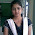Before starting this lecture, I'm assuming that you are familiar with Loops in programming language and can use it easily. If you do not then click here before starting with arrays.

Now we start the lecture.

Whenever we want to store data in our program we declare a variable of a particular type and store data in it. Just like if we want to store a number/integer we must declare a variable of int and store the data in it. Just like
int a = 5;
Now the variable a has value of 5

But if we want to store 100 different numbers in our program then what? If we declare different variables a, b, c, d,....... up to our requirement. Then this would be considered as very bad programming. Let if we did this and we want to get numbers from the user or want to print all 100 values that are in different variables, then we have to write 100 variables name and we have to remember all variable names. The code will also become complex to handle.

To overcome this situation arrays were introduced in the programming language. We simply declare an array of particular size and all the values are under one name with different indexes, I will explain how it works.

## What is Array?

An array is the data structure consisting of related data items of the same type.

So, an array is the related data item of the same type. One can say "What are related data items? " I would say please wait until the lecture ends.

Now, if we want to store 5 different integers in our program we simply declare an array of integer just like
int myFirstArray;
When the upper line runs 5 blocks with the same name (Array name) but different indexes will form in the memory that can store integers in it. As shown in the figure
Here, myFirstArray is the name of the array we declare (you can use any name) and square brackets show that this is not a simple variable of an integer but an array of type integer and in square brackets 5 shows that we can store 5 different integers in this array by using indexes from 0 to 4.

To access any block of the array we just need the array name and the index of the block in the square bracket.

Array index always starts from 0 and ends at size-1. We can store elements of the same type of which we declare an array. We have declared an array of the integer of size 5. So we can store values just like
myFirstArray = 56;
myFirstArray = 6;
myFirstArray = 10;
myFirstArray = 25;
myFirstArray = 1;
When this code runs the array will have values in their blocks just like as shown in the figure

All the values are under one name with different indexes. We just change indexes to access any value in our array. (If an array is of size hundred we don't need to remember hundred variable names but just one name which is of the array name.)

If we want to print 25 from our array we can use statement just like
printf("Value at index 3: %d", myFirstArray);
This will print the value of index 3 just like
Value at index 3: 25

If our array is of small size, we can print and get values manually but this does not actually benefit us. So we use loops for this.
If we want to print our array we have declared and initialized by using loop we just write three lines of code just like

int i;

for(i=0 ; i<=4 ; i++){

printf("Value at index %d: %d \n",i, myFirstArray[i]);

}
Output:
Value at index 0: 56
Value at index 1: 6
Value at index 2: 10
Value at index 3: 25
Value at index 4: 1

If we want to get values from the user in our array we have declared by using loop we simply write for this also

int i;

for(i=0 ; i<=4 ; i++){

printf("Enter Value at index %d : ",i);
scanf("%d", &myFirstArray[i]);

}

This will get 5 values from the user.

Here the main benefit is that, if we want to get 10 or 100 values from the user our code will remain same, you only need to change the size in the declaration and condition in the loop, nothing else and this will work for more values. So the code will remain relatively small.

In defining, the term related data items are used. why this is used? This tells that we use an array where the whole data are related to each other. For example, marks of a student etc.

Now you would have a better understanding of arrays, how it works and why to use arrays etc.

You can also use Multidimensional arrays in C Programming.

1.2.